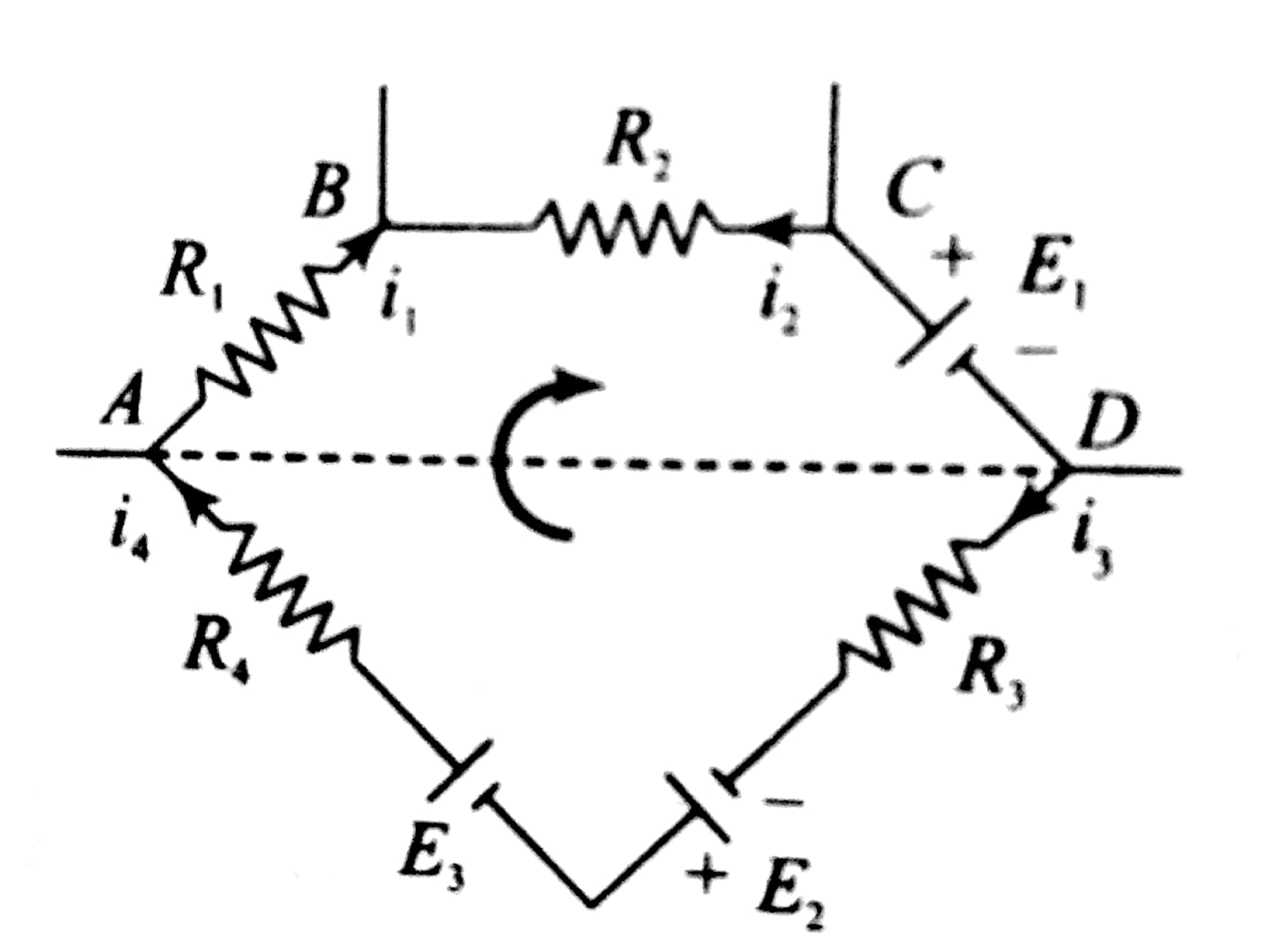## Filters

QIn the circuit shown, the current in the 1resistor is :

• Option 1)

1.3 A ,from P to Q

• Option 2)

0 A

• Option 3)

0.13 A ,from Q to P

• Option 4)

0.13 A ,from P to Q

111 Views
N

answer is option 3)0.13A from Q  to P

•  Let, Current i1 from 6v battery passes through 3 ohm resistance . At Q current breaks and current i2 goes through 3 ohm and ( i1-i2 ) goes through 1 ohm ,.
• Applying, kirchoffs law in 1st and 2nd loop,we get two eqns as follows
• 4i1-i2=6
• 6i2-i1=9
• solving ,,  i1-i2 =0.13 A

As we discussed in

In closed loop -

- whereinApplying KVL in loop PQCDP

-1I2-3I2+9-2I2+-1I1=0

6I2-I1=9-(i)

Applying KVL in loop PQBAP

4I1-I2=6-(ii)

from equation (i) and (ii) we get

the current in the 1 resistor is 0.13 A from Q to P

Option 1)

1.3 A ,from P to Q

Option 2)

0 A

Option 3)

0.13 A ,from Q to P

Option 4)

0.13 A ,from P to Q

Exams
Articles
Questions